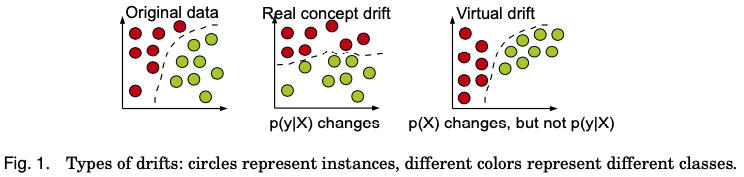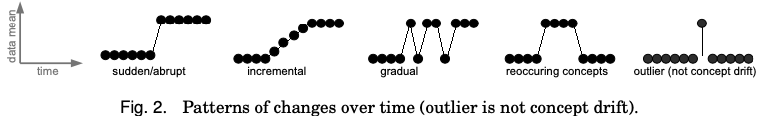Data Bytes - Concept Drift

Ler em 🇧🇷

Data Bytes is a blog series that consists of small posts (or bytes) that elaborates a subject in a summarized way - almost like an introduction to incite you to learn more about it.

Today we are going to talk a little bit about concept drift!

Concept Drift

Before we start, let’s define a few premisses from which we are going to elaborate: first, we’ll be looking at a supervised learning problem - from which we predict a target variable $y$ given a set of input variables $X$.

For classification tasks (where $y$ is a categorical value), we can describe this scenario using the Bayesian Decision Theory (DUDA et al, 2001):

$p(y|X) = \dfrac{p(y)p(X|y)}{p(X)} \\ \text{where} \\ p(X)=\sum^{c}_{y=1}p(y)P(X|y)$

Here, our classification probability $p(y|X)$ is described by the prior probabilities of the classes $p(y)$ and the class conditional probability density functions $p(X|y)$ (is worth mentioning that we are assuming equal costs of misclassification for $p(X)$).

For most machine learning problems (or the most classical scenarios) we are given a dataset (an historical and labeled dataset, consisting with $(X_{n}, y_{n})$) from which we learn from and develop a model (Gama et al (2013) usually refers to this as the offline scenario). For an online consumption scenario, this model receives data in a potentially infinite stream for making predictions (‘mapping’ the input space X for its corresponding output y).

In a real-world scenario the data is expected to evolve over time, meaning non-stationarity: our environment is dynamic and is constantly changing in time.

What is Concept Drift?

OK, now that we have seen a little bit the scenario we are going to be approaching we can proceed to understanding what concept drift is.

Gama et al (2013) defines concept drift as:

$\exists X:p_{t_{0}}(X,y) \ne p_{t_{1}}(X,y)$

Here, $p_{t_{n}}$ denotes the joint distribution at time $t_{n}$ between our input variables $X$ and our target $y$. In this definition we are arguing that because of our non-stationary environment, there exists a time $t$ where our joint probability $p(X,y)$, learned from our training dataset, does not corresponds to the real distribution anymore.

Considering this scenario, the following event may happen: our priors probabilities $p(y)$ change and/or our class conditional probabilities $p(X|y)$ change, resulting in a change on our posterior probabilities of classes $p(y|X)$, where our predictions may not hold anymore.

Types of Concept Drift

There are different types of concept drifts that can arrive in the changes above:

Real Concept Drift

This is also known as concept shift or conditional change and refers to changes in $p(y|X)$, i.e. changes on our posterior probability that could arrive with changes in the distribution of our input data P(X) or not.

One thing to mention is that a real concept drift scenario is very hard to notice, especially if you’re in a scenario where your true feedback labels are delayed, that is, the response for a right or wrong prediction for the model only arrive after a time window.

Virtual Drift

This is also known as feature change, sampling drift, or temporary drift and refers to a change in our input data distribution $P(X)$ without affecting our posterior probability distribution $p(y|X)$.

Changes in the input distribution are easier to detect, especially because true feedback labels are not needed in this scenario, you can compare distributions from input variables from your training dataset and your ‘online dataset’ (there are many more ways to detect virtual drifts).

Visual Comparison

Gama et al (2013) provides a very intuitive visual comparison of real and virtual drifts:You can see that in the case of a real concept drift, the decision boundary changed, meaning that predictions obtained from the ‘original data decision boundary’ will not hold anymore; by the other hand, you can see that in the case of a virtual drift, the original decision boundary still holds, even with the change in the input distribution data.

Natures of Change

We saw that the key of understanding concept drift (real or not) is that real-world scenarios are not stationary, meaning that changes will happen.

Changes have many forms, and in order to understand these changes it may be interesting to understand its causes and their rates.

Causes of Change

As we saw previously, our scenario consists of a model learning from previous annotated observations, with a finite set of input variables (features). When developing a model we usually perform many analysis (from a statistical or business nature) to understand which variables should be used to train a model.

Nevertheless, many hidden variables influence a target variable and they could be missing for any reasons, from not being yet available (are observable) to are not observable. Still, one possible cause of change is when a hidden variable distribution change, and as a result concepts learned previously don’t hold anymore.

Another possible cause of change is when the nature of a concept change, this is more related to a ‘business’ view of the problem and it may be related to policy, market, or even vision changes in your business.

Rates of Change

In my opinion, this is a hot topic, especially because there is a little confusion on what concept drift and how to observe them. Gama et al (2013) provides us a nice visual representation on different patterns of change on a one-dimension data:Here we can see different patterns of change, some cases are easier to detect (sudden/abrupt or gradual) changes, other are more difficult (incremental), or some of them may be related to ‘seasonal’ behaviours (reoccurring concepts), and some of them are not actually drifts (like outliers or anomalies).

The real challenge here is how to detect real concept drift from noise errors or anomalies.

Conclusion

This data bytes post introduced a little bit on what concept drift is and on possible challenges when trying to detect it. The truth is that detecting concept drift is not trivial and we may have to rely on different proxies when trying to spot a situation where concept drift emerged - this is especially difficult when dealing with delayed feedback outputs (true labels).

The term of concept drift is also a hot topic when we talk about Active Learning, and most of the references bellow were actually written on this subject (Joao da Gama is one of my greatest references for this subject, so be sure to check it out!).

LAZARESCU, M.; VENKATESH, S.; BUI, HH. Using multiple windows to track concept drift. 2004. Available at: https://www.researchgate.net/publication/220571442_Using_multiple_windows_to_track_concept_drift

GAMA, J.; ZLIOBAITE, I.; BIFET, AL.; PECHENIZKIY, M.; BOUCHACHIA, A. A Survey on Concept Drift Adaptation. 2013. Available at: https://dl.acm.org/doi/10.1145/2523813

GAMA, J.; AGUILAR-RUIZ J. S.; KLINKENBERG, R. Knowledge Discovery from Data Streams. 2010. Available at: https://www.researchgate.net/publication/220571629_Knowledge_discovery_from_data_streams

PAKA, A. How to detect model drift in ML Monitoring. Available at: https://blog.fiddler.ai/2020/08/how-to-detect-data-drift/